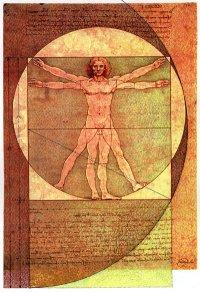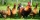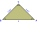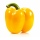# Ratio

6 numbers are in the ratio 1:5:1:5:5:5. Their sum is 242. What are the numbers?

Correct result:

a =  11
b =  55
c =  11
d =  55
e =  55
f =  55

#### Solution:

$a = \dfrac{ 1 \cdot 242}{ 1+5+1+5+5+5} = 11$
$b = \dfrac{ 5 \cdot 242}{ 1+5+1+5+5+5} = 55$
$c = \dfrac{ 1 \cdot 242}{ 1+5+1+5+5+5} = 11$
$d = \dfrac{ 5 \cdot 242}{ 1+5+1+5+5+5} = 55$
$e = \dfrac{ 5 \cdot 242}{ 1+5+1+5+5+5} = 55$
$f = \dfrac{ 5 \cdot 242}{ 1+5+1+5+5+5} = 55$Our examples were largely sent or created by pupils and students themselves. Therefore, we would be pleased if you could send us any errors you found, spelling mistakes, or rephasing the example. Thank you!

Please write to us with your comment on the math problem or ask something. Thank you for helping each other - students, teachers, parents, and problem authors.Tips to related online calculators
Check out our ratio calculator.
Do you have a system of equations and looking for calculator system of linear equations?

## Next similar math problems:

• New ratioThe ratio of ducks and chicken in our yard is 2 : 3. The total number of ducks and chickens together is 30. Mother gave 3 of the chickens to our neighbor. What is the new ratio now?
• SummandsWe want to split the number 110 into three summands so that the first and the second summand are in the ratio 4: 5, and the third with the first are in ratio 7: 3. Calculate the smallest of the summands.
• The circumferenceThe circumference and width of the rectangle are in a ratio of 5: 1. its area is 216cm2. What is its length?
• Conical bottleWhen a conical bottle rests on its flat base, the water in the bottle is 8 cm from it vertex. When the same conical bottle is turned upside down, the water level is 2 cm from its base. What is the height of the bottle?
• Flowers 2Cha cruz has a garden. The ratio roses to tulips is 2 : 5, the ratio of roses to orchids is 7 : 6. Cha cruz wonders what the ratio of tulips to orchids is. If Cha cruz has 183 plants, how many of each kind are there?
• Isosceles triangleIn an isosceles triangle, the length of the arm and the length of the base are in ration 3 to 5. What is the length of the arm?
• In aIn a triangle, the aspect ratio a: c is 3: 2 and a: b is 5: 4. The perimeter of the triangle is 74cm. Calculate the lengths of the individual sides.
• Vegetable mealThe cook was doing meal - in ratio 4: 3: 1 mix tomatoes: pepper: onion. Onions were 5 kg less than peppers. How many kgs of tomatoes did he need to prepare the meal?
• Trio ratioHans, Alena and Thomas have a total of 740 USD. Hans and Alena split in the ratio 5: 6 and Alena and Thomas in the ratio 4: 5. How much will everyone get?
• Gasoline-oil ratioThe manufacturer of a scooter engine recommends a gasoline-oil fuel mixture ratio of 15 to 1. In a particular garage, we can buy pure gasoline and a gasoline-oil mixture, which is 75% gasoline. How much gasoline and how much of the gasoline-oil mix do w
• Two diggersTwo diggers should dig a ditch. If each of them worked just one-third of the time that the other digger needs, they'd dig up a 13/18 ditch together. Find the ratio of the performance of this two diggers.
• Boys to girlsThe ratio of boys to girls in a party is 3:5 . If 6 more boys arrived and 4 girls left the party, the ratio of boys to girls would be 5:6 . How many are in the party originally?
• Ratio of sidesThe triangle has a circumference of 21 cm and the length of its sides is in a ratio of 6: 5: 3. Find the length of the longest side of the triangle in cm.
• PupilsThere are 32 pupils in the classroom, and girls are two-thirds more than boys. a) How many percents are more girls than boys? Round the result to a whole percentage. b) How many are boys in the class? c) Find the ratio of boys and girls in the class. W
• The towerThe observer sees the base of the tower 96 meters high at a depth of 30 degrees and 10 minutes and the top of the tower at a depth of 20 degrees and 50 minutes. How high is the observer above the horizontal plane on which the tower stands?
• Rectangular plotThe dimensions of a rectangular plot are (x+1)m and (2x-y)m. If the sum of x and y is 3m and the perimeter of the plot is 36m. Find the area of the diagonal of the plot.
• ParkingAt a building parking, 245 spaces are for cars, 56 are for vans and the rest are for buses. If 14% of all parking spaces are for buses, how many parking spaces are there in the building? How many spaces are for buses?